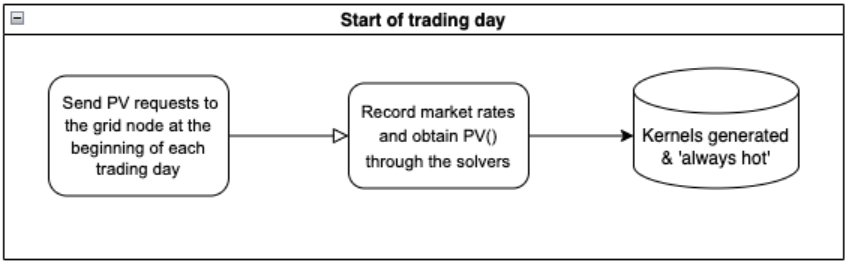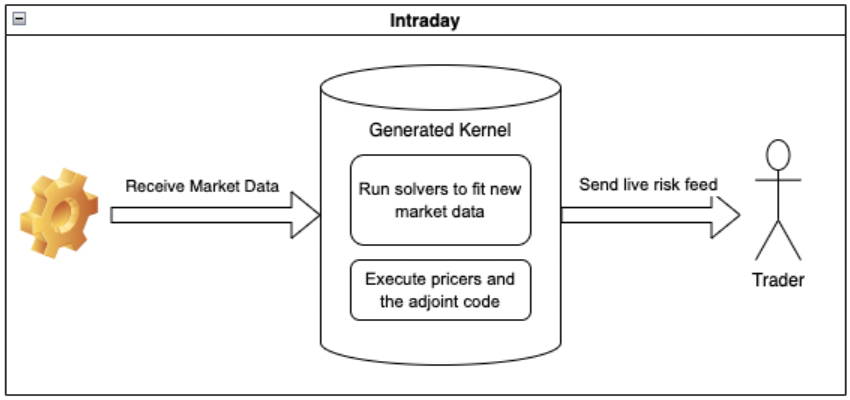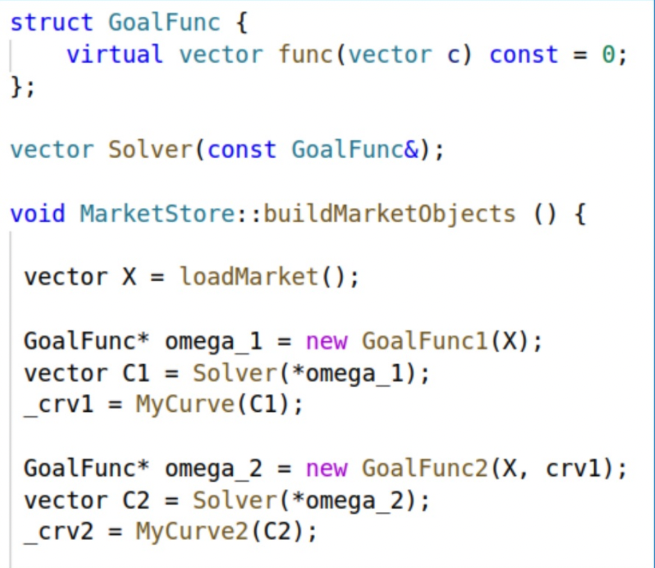# Blog Post

## How to Transition from Batch Risk to Real-time Risk

A recipe to transition to Live Risk using a modern Automatic Adjoint Differentiation (AAD) tool in conjunction with the Automated Implicit Function Theorem technique

## Introduction

Batch processing for portfolio risk calculation has been a standard for decades with risk reports being produced overnight. But now, traders want to see their risk in real-time, and quant libraries that are used in practice were not designed for this.

Batch execution is a typical process for producing detailed risk reports. This mode of execution is sensible for the bump-and-revalue, brute force method, of computing risks and scenario analysis. Using our modern AAD tool, risk management can be taken to the next level - seeing risk "live", and having your risk reports update almost instantly with every new market tick. Traditional batch execution involves breaking down the trade portfolio into jobs, serialising, and de-serialising for execution on the grid, and, eventually, carrying out the jobs in parallel. Batch execution usually takes several hours overnight, whereas live risk can give you results in a fraction of the time, enabling faster decision-making and giving you significant competitive advantage, especially in turbulent intraday trading times.

Batch processing is slow - and dangerous. When systems are slow, people cut corners, and don’t compute all scenario risks, leaving some risks unmonitored. This was demonstrated in exotic derivatives when Korean autocallable notes pushed over a trillion Won (\$810 million) of South Korean equity-linked securities into the red in Q3 of 2022.

Transitioning into live risk is much desired as it can yield better returns to stakeholders, reduce operational risks as well as improve regulatory compliance. However, delivering such functionality typically needs major investment as it requires rewriting large parts of the quant library.

In this post, we present an alternative way to transition from overnight risk calculations to live risk without embarking on a multi-year IT transformation project. We show how the Automated Implicit Function Theorem (AIFT) and a modern AAD tool can be used in a real production code environment to achieve an ‘always on’ Risk Server, and we outline the steps required to transition.

## Example (and we can show how this can be applied to your quant library)

Let’s start with an example of QuantLib - a seasoned real-life library that we integrated with a modern AAD tool - ‘MatLogica AADC’. First, we implement interest rate curve fitting using the Levenberg-Marquardt multidimensional optimization method followed by repricing an IR swap portfolio. Then we transform analytical risk w.r.t. the calibrated curve values into tradeable market rates via AIFT. Using a synthetic market data feed, we price a portfolio of 1000 12-year interest rate swaps with random start/stop dates and frequencies. The whole process of pricing and risk, including curve fitting, takes 20ms for a new market data update on a single budget CPU. You can play with this demo on MatLogica.com by clicking the Launch The Live Risk App:
We can now see how the existing batch-mode analytics can be transitioned to live risk, making your traders happy!

## The Magic behind the Live Risk

The Implicit Function Theorem (IFT) is a powerful tool for calculating derivatives of functions that solve inverse problems i.e. calibration. It is commonly believed that a degree of manual intervention is required to enable financial code to take advantage of Automatic Adjoint Differentiation (AAD) when analytics include model calibrations (implicit functions) solved by minimizers/solvers. However, in 2022, Dmitri Goloubentsev, Evgeny Lakshtanov, and Vladimir Piterbarg published an article "Automatic Implicit Function Theorem'' in Risk.NET that proposes a smart way to apply the IFT, enabling fully-automated differentiation of exact-fit calibration routines and providing an approximate solution to nearly-exact calibration problems. This approach can greatly simplify and automate AAD integration into a legacy quant library and turn it into a high-performance intraday risk system.

The key to Live risk is performance, so a high-performing AAD tool is required. Matlogica’s AADC library achieves the adjoint factor of less than 1, meaning that all the risks can be computed faster than the PV. Therefore, it is a great fit. The performance is achieved by recording the computation once and producing a highly-optimised and vectorised ‘kernel’ to use for repeated calculations.

## Proposed Software Architecture

A high-level system functionality can be described as follows: at the beginning of the trading day, MatLogica's AADC generates binary ‘kernels’ for the forward and adjoint execution that will be in an ‘Always hot’ state on the grid:During the trading day, the kernel receives market data updates, runs the solvers to fit the market data, and executes prices along with the adjoint code. The prices and risks will be sent to the traders:So now, having looked at the overall approach, let’s see the maths behind it.

## The Math Behind it

#### 1. Set Up

Suppose that we have an objective function $\Omega \left(X,C\right)$ where $X\left(x\right)$ stands for the market observable data in the market variables $x$ and $C$ stands for the coefficients of the model. We calibrate the coefficients to the market data by solving the equation
$\Omega \left(X,C\right)=0$(1)
Then the calibrated coefficients $C\left(x\right)$ can be used to compute another value of interest $U\left(x\right)=V\left(C\left(x\right)\right)$ that could be the price of the assets, XVA, etc.
We know pretty well how to solve the forward problem of computing $U\left(x\right)$ (by using Newton solvers, Levenberg-Marquardt, etc.), let us focus on backpropagating the sensitivities with respect to the market data $x$ (representing the rates, spreads, etc.). Explicitly, we need to compute (this is (12)):$\frac{\partial U}{\partial x}=-\frac{\partial V}{\partial c}{\left(\frac{\partial \Omega }{\partial C}\right)}^{-1}\frac{\partial \Omega }{\partial x}.$
In this formula, $\frac{\partial V}{\partial c}$ can be obtained with a straightforward application of AAD, and $\frac{\partial \Omega }{\partial c}$ is usually already computed during the forward step (if using Newton solvers or Levenberg-Marquardt). So we need to focus on computing $\frac{\partial \Omega }{\partial x}$. Again, in this simple calibration example (1), this is straightforward.

#### 2. Calibration in steps

In practice, calibration is often done by using multiple solvers executed in a sequence. So instead of one formula (1) we get multiple calibration steps
$\begin{array}{cc}& {\Omega }_{1}\left({C}_{1}\right)=0\\ & {\Omega }_{2}\left({C}_{2}\right)=0\\ & \dots \\ & U\left(x\right)=V\left({C}_{1},{C}_{2},\dots \right)\end{array}$
Here the multiple calibration steps ${\Omega }_{i}$solve lower-dimensional calibration problems. The issue is that the dependence on $X$ and ${C}_{j},1\le j in ${\Omega }_{i}$ is often implicit (i.e. they are hidden variables) since the abstract virtual interface that one uses to implement Newton solvers and Levenberg-Marquardt may hide these dependencies. See Figure 1 for a code example on how a virtual function GoalFunc2 (an implementation for ${\Omega }_{2}$) can't see a dependence on $X$ and ${C}_{1}$. In practice, the issue may be even more severe as GoalFunc may be hidden deep inside the library.#### 2.1 Naive IFT

Due to the hidden variables, a naive application of AAD to compute $\frac{\partial \Omega }{\partial c}$ would often require a lot of refactoring of the existing code to track the dependencies. Writing ${W}_{i}$ for the hidden depedencies in ${\Omega }_{i}$, one would need to identify the hidden variables ${W}_{i}$ and compute the Jacobians $\frac{\partial {\Omega }_{i}}{\partial {W}_{i}}$ of the functions ${\Omega }_{i}\left({C}_{i},{W}_{i}\right)$. Note that these Jacobians can be very large.

#### 2.2 AIFT

Suppose that we have just two calibration steps and the calibration function $\Omega \left({C}_{2},{W}_{2}\right)$ depends on the hidden variables ${W}_{2}$. We want to backpropagate $U\left(x\right)=V\left({C}_{1},{C}_{2}\right)$. The intuition behind the AIFT approach is as follows. When we are using AAD to compute the derivatives, executing $adjV\left(\right)$ calculates $adj{C}_{2}$. Executing $adj{\Omega }_{2}\left(\right)$ then updates $adj{C}_{2}$ and $adj{W}_{2}$. In this process, we are free to choose $adj{\Omega }_{2}$. The idea is to choose $adj{\Omega }_{2}$ in such a way that the adjoint update cancels out the value of $adj{C}_{2}$ and makes $adj{C}_{2}=0$. This will propagate $adj{W}_{2}$ automatically. The correct choice for $adj{\Omega }_{2}$ that achieves this is (see (20)):
$adj{\Omega }_{2}:=-\frac{\partial V}{\partial {C}_{2}}{\left(\frac{\partial {\Omega }_{2}}{\partial {C}_{2}}\right)}^{-1}$
Note from the discussion at the end of Section 1 that both quantities in the product are readily available. This allows integrating AAD by using checkpointing without going into the solvers or identifying the hidden variables. For multiple calibration steps, one would do the same thing at every level of the calibration process.

## Conclusions

To summarize, the AIFT approach offers the following benefits:
• Straightforward automatic handling for solvers and minimizers with no refactoring of the codebase.
• The computation of $\frac{\partial {\Omega }_{i}}{\partial {W}_{i}}$ is not required which leads to a much faster backpropagation.

By using Matlogica's AADC library, a batch system can be transformed into an ‘always on’ Risk Server without embarking on a multi-year transformation project. To achieve this, the following steps need to be implemented: Integrate Matlogica's AADC into your existing Quant library.

1. Send PV requests to the grid node at the beginning of each trading day.
2. Record market rates and obtain $PV\left(\right)$ through the solvers.